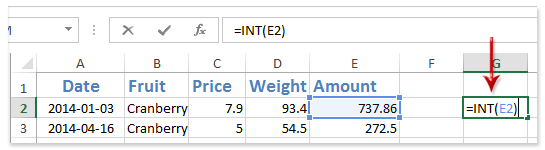I have an excel formula that is producing a lot of decimal places and I cannot reduce them using the format cell -> numbers -> decimal places options. Here is the formula.

Cell named V01_MIN V01_MIN =MIN(6:6) Has a value of 2

Cell named V01_MAX V01_MAX =MAX(6:6) Has a value of 1800

Cell named V01_A V01_A =1-V01_MIN*V01_B Has a value of 0.889877642

Cell named V01_B V01_B =99/(V01_MAX-V01_MIN) Has a value of 0.055061179

```X6=723
X7=V01_A+V01_B*X6 (value of 40.69911012)
X8=1
X9=X7*X8 (value of 40.69911012)
X10=1
X11=X9*X10 (value of 40.69911012)
X13==CONCATENATE(X12,", ",X11)
```

The final results of X13 are:

```V01, 1162, 40.6991101223582
```

I want them to be:

```V01, 1162, 40.7
```

I'm trying to figure out how to make this happen. I've already tried changing the cell formatting on all of these cells (including the final cell) to one decimal palce and that didn't work.Mar 23 in Database 46 views

## 1 answer to this question.

1) E2 is the cell from which you will subtract decimal digits in the formula =INT(E2).
2) After a decimal, the INT function can keep particular digit positions. You can use the formula =INT(E2*10)/10 to delete all digits following the decimal save the first one.

In a blank cell besides the value you will remove digits after decimal, enter the formula =INT(E2), and the drag the Fill Handle to the range you need.answered Mar 24 by
• 8,820 points

## Excel formula to remove comma, spaces, period and add a text

The steps to accomplish this are as ...READ MORE

## Remove time from date field in Excel formula

Use the Find And Replace function to ...READ MORE

## Excel formula to remove space between words in a cell

There are three fast techniques to get ...READ MORE

## Group by Sum in Excel

It is very easy and you can ...READ MORE

## Excel - Split cell with a delimiter and if delimiter not found get complete cell value to another cell

Select the row or column that you ...READ MORE

## Remove duplicates within a row

To check for duplicates, choose the cells ...READ MORE

## EXCEL: How to automatically add serial number in Excel Table using formula that is immune to filtering / sorting?

That's a great question, and it's a ...READ MORE